# 机器学习【十】模型评估与优化

• 使用交叉验证对模型进行评估
• 使用网格搜索寻找模型的最优参数
• 对分类模型的可信度进行评估

1.sklearn中的交叉验证法

sklearn中默认使用的是 K折叠交叉验证法：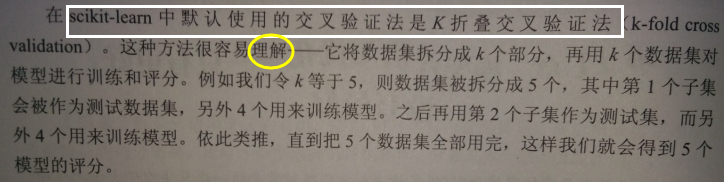#导入红酒数据集
#导入交叉验证工具
from sklearn.model_selection import cross_val_score
#导入用于分类的支持向量机模型
from sklearn.svm import SVC
#载入红酒数据集
#设置SVC的核函数为linear
svc = SVC(kernel='linear')
#使用交叉验证法对SVC进行评分
scores = cross_val_score(svc,wine.data,wine.target)
#得分
print(scores)
[0.83333333 0.95       1.        ]

【结果分析】

先导入了scikit_learn的交叉验证评分类，然后使用SVC对酒的数据集进行分类，默认情况下，cross_val_score会使用3个折叠，因此，会得到3个分数

#使用.mean() 获得分数的平均值
print(scores.mean())
0.9277777777777777

【结果分析】

交叉验证法平均分为0.928分

#设置cv参数为6
scores = cross_val_score(svc,wine.data,wine.target,cv=6)
print(scores)
[0.86666667 0.9        0.93333333 0.96666667 1.         1.        ]

print(scores.mean())
0.9444444444444445

【结果分析】

在sklearn中，cross_val_score对于分类模型默认使用的是K折叠交叉验证，而对于分类模型则默认使用分层K交叉验证法

#打印红酒数据集的分类标签
print(wine.target)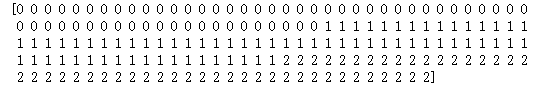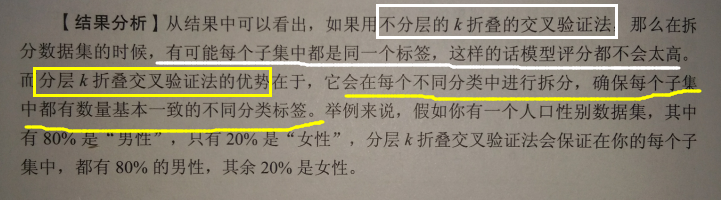2.随机拆分和“挨个儿试”

随机拆分原理——先从数据集中随机抽一部分数据作为训练集，再从其余的部分随机抽一部分作为测试集，进行评分后再迭代，重复上一步操作，直到把我们希望的迭代次数全跑完

#导入随机拆分工具
from sklearn.model_selection import ShuffleSplit
#设置拆分的数为10个
shuffle_split = ShuffleSplit(test_size=.2,train_size=.7,n_splits=10)
#对拆分好的数据进行交叉验证
scores = cross_val_score(svc,wine.data,wine.target,cv=shuffle_split)
print(scores)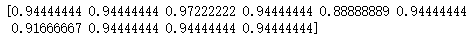【结果分析】

ShuffleSplit一共为SVC模型进行了10次评分，最终得分即10个评分的平均值

#导入LeaveOneOut
from sklearn.model_selection import LeaveOneOut
#设置cv参数为leaveoneout
cv = LeaveOneOut()
#重新进行交叉验证
scores = cross_val_score(svc,wine.data,wine.target,cv=cv)
print('迭代次数：',len(scores))
print('平均分:',scores.mean())
迭代次数： 178

【结果分析】

由于酒的数据集中有178个样本，所以迭代了178次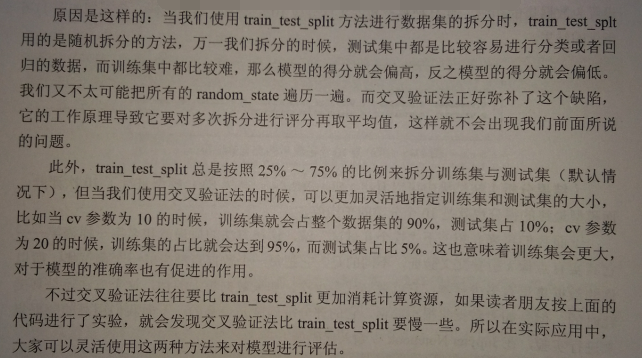使用网格搜索优化模型参数

1.简单网格搜索

#导入套索回归模型
from sklearn.linear_model import Lasso
#导入数据集拆分工具
from sklearn.model_selection import train_test_split
X_train,X_test,y_train,y_test = train_test_split(wine.data,wine.target,random_state = 38)
#设置初始分数为0
best_score = 0
#设置alpha的参数遍历0.01，0.1，1，10
for alpha in [0.01,0.1,1,10]:
#最大迭代数遍历100，1000，5000，10000
for max_iter in [100,1000,5000,10000]:
lasso = Lasso(alpha=alpha,max_iter=max_iter)
#训练套索回归模型
lasso.fit(X_train,y_train)
score = lasso.score(X_test,y_test)
#令最佳分数为所有分数中的最高值
if score >best_score:
best_score = score
#定义字典，返回最佳参数和最佳迭代数
best_parameters={'alpha':alpha,'最大迭代数':max_iter}
print('最高分：',best_score)
print('最佳参数设置',best_parameters)
最高分： 0.8885499702025688

【结果分析】

快速找到了~~

举例：

#导入套索回归模型
from sklearn.linear_model import Lasso
#导入数据集拆分工具
from sklearn.model_selection import train_test_split
X_train,X_test,y_train,y_test = train_test_split(wine.data,wine.target,random_state = 0)
#设置初始分数为0
best_score = 0
#设置alpha的参数遍历0.01，0.1，1，10
for alpha in [0.01,0.1,1,10]:
#最大迭代数遍历100，1000，5000，10000
for max_iter in [100,1000,5000,10000]:
lasso = Lasso(alpha=alpha,max_iter=max_iter)
#训练套索回归模型
lasso.fit(X_train,y_train)
score = lasso.score(X_test,y_test)
#令最佳分数为所有分数中的最高值
if score >best_score:
best_score = score
#定义字典，返回最佳参数和最佳迭代数
best_parameters={'alpha':alpha,'最大迭代数':max_iter}
print('最高分：',best_score)
print('最佳参数设置',best_parameters)
最高分： 0.8298747376836272

【结果分析】

稍微对train_test_split拆分数据集的方式做一点变更，最高分酒降到了0.83

2.与交叉验证结合的网格搜索

#导入numpy
import numpy as np
#设置alpha的参数遍历0.01，0.1，1，10
for alpha in [0.01,0.1,1.0,10.0]:
#最大迭代数遍历100，1000，5000，10000
for max_iter in [100,1000,5000,10000]:
lasso = Lasso(alpha=alpha,max_iter=max_iter)
scores = cross_val_score(lasso,X_train,y_train,cv=6)
score = np.mean(scores)
#令最佳分数为所有分数中的最高值
if score >best_score:
best_score = score
#定义字典，返回最佳参数和最佳迭代数
best_parameters={'alpha':alpha,'最大迭代数':max_iter}
print('最高分：',best_score)
print('最佳参数设置',best_parameters)
最高分： 0.8652073211223437

【结果分析】

这里我们做了一点手脚，就是只用先前拆分好的X_train来进行交叉验证，以便于我们找到最佳参数后，再用来拟合X_test 来看一下模型的得分

#用最佳参数模型拟合数据
lasso = Lasso(alpha=0.01,max_iter=100).fit(X_train,y_train)
print('数据集得分：',lasso.score(X_test,y_test))
数据集得分： 0.819334891919453

【结果分析】

#导入网格搜索工具
from sklearn.model_selection import GridSearchCV
#将需要遍历的参数定义为字典
params = {'alpha':[0.01,0.1,1.0,10.0],'max_iter':[100,1000,5000,10000]}
#定义网格搜索中使用的模型和参数
grid_search = GridSearchCV(lasso,params,cv=6)
#使用网格搜索模型拟合数据
grid_search.fit(X_train,y_train)
print('模型最高分:',grid_search.score(X_test,y_test))
print('最优参数：',grid_search.best_params_)
模型最高分: 0.819334891919453

【结果分析】

GridSearchCV中的best_scores_ 属性，会存储模型在交叉验证中所得的最高分，而不是测试集上的得分

#打印网格搜索中的best_score_属性
print('交叉验证最高分：',grid_search.best_score_)
交叉验证最高分： 0.8653192931146032

【结果分析】

这里的得分和cross_val_score得分是完全一致的，说明GridSearchCV 本身就是将交叉验证和网格搜索封装一起的方法

GridSearchCV虽然强悍，但需要反复建模——> 所需要的计算时间往往更长

1.分类模型中的预测准确率

在sklearn中，很多用于分类的模型都有一个 predict_proba功能——用于计算模型在对数据集进行分类时，每个样本属于不同分类的可能性是多少

#导入数据集生成工具
from sklearn.datasets import make_blobs
#导入画图工具
import matplotlib.pyplot as plt
#生成样本数为200，分类为2，标准差为5的数据集
X,y = make_blobs(n_samples=200,random_state=1,centers=2,cluster_std =5)
#绘制散点图
plt.scatter(X[:,0],X[:,1],c=y,cmap=plt.cm.cool,edgecolor='k')
plt.show()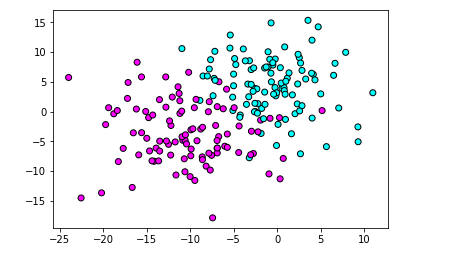【结果分析】

#导入高斯贝叶斯模型
from sklearn.naive_bayes import GaussianNB
X_train,X_test,y_train,y_test = train_test_split(X,y,random_state=68)
#训练高斯贝叶斯模型
gnb = GaussianNB()
gnb.fit(X_train,y_train)
#获得高斯贝叶斯的分类准确概率
predict_proba = gnb.predict_proba(X_test)
print('预测准确率形态：',predict_proba.shape)
预测准确率形态： (50, 2)

【结果分析】

#打印准确概率的前5个
print(predict_proba[:5])
[[0.98849996 0.01150004]
[0.0495985  0.9504015 ]
[0.01648034 0.98351966]
[0.8168274  0.1831726 ]
[0.00282471 0.99717529]]

【结果分析】

反应的是测试集前5个样本的分类准确率

#设定横纵轴范围
x_min,x_max= X[:,0].min()-.5,X[:,0].max()+.5
y_min,y_max= X[:,1].min()-.5,X[:,1].max()+.5
xx,yy = np.meshgrid(np.arange(x_min,x_max,0.2),np.arange(y_min,y_max,0.2))
Z = gnb.predict_proba(np.c_[xx.ravel(),yy.ravel()])[:,1]
Z = Z.reshape(xx.shape)
#绘制等高线
plt.contourf(xx,yy,Z,cmap=plt.cm.summer,alpha=.8)
#绘制散点图
plt.scatter(X_train[:,0],X_train[:,1],c=y_train,cmap=plt.cm.cool,edgecolor='k')
plt.scatter(X_test[:,0],X_test[:,1],c=y_test,cmap=plt.cm.cool,edgecolor='k',alpha=0.6)
#设置横纵轴范围
plt.xlim(xx.min(),xx.max())
plt.ylim(yy.min(),yy.max())
#设置横纵轴的单位
plt.xticks(())
plt.yticks(())
plt.show()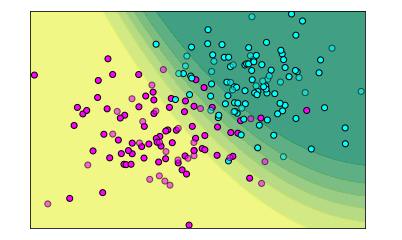【结果分析】

圆点代表样本数据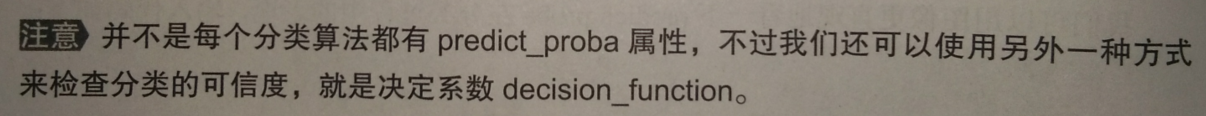2.分类模型中的决定系数

同预测准确率类似，决定系数decision_function 也会给我们返回一些数值——告诉我们模型认为某个数据点处于某个分类的“把握”有多大

不同的是，在二元分类任务中，只返回一个值——正数，属于分类1；负数，属于分类2

#导入SVC模型
from sklearn.svm import SVC
#使用训练集训练模型
svc = SVC().fit(X_train,y_train)
#获得SVC的决定系数
dec_func = svc.decision_function(X_test)
#打印决定系数中的前5个
print(dec_func[:5])
[ 0.02082432  0.87852242  1.01696254 -0.30356558  0.95924836]

#使用决定系数绘图
Z = svc.decision_function(np.c_[xx.ravel(),yy.ravel()])
Z = Z.reshape(xx.shape)
#绘制等高线
plt.contourf(xx,yy,Z,cmap=plt.cm.summer,alpha=.8)
plt.scatter(X_train[:,0],X_train[:,1],c=y_train,cmap=plt.cm.cool,edgecolor='k')
#绘制散点图
plt.scatter(X_test[:,0],X_test[:,1],c=y_test,cmap=plt.cm.cool,edgecolor='k',alpha=0.6)
plt.title('SVC decision_function')
#设置横纵轴范围
plt.xlim(xx.min(),xx.max())
plt.ylim(yy.min(),yy.max())
#设置横纵轴的单位
plt.xticks(())
plt.yticks(())
plt.show()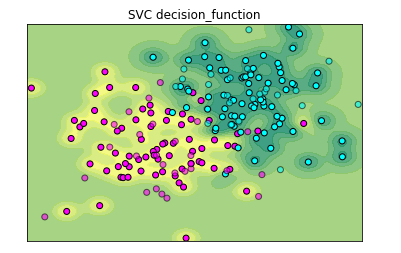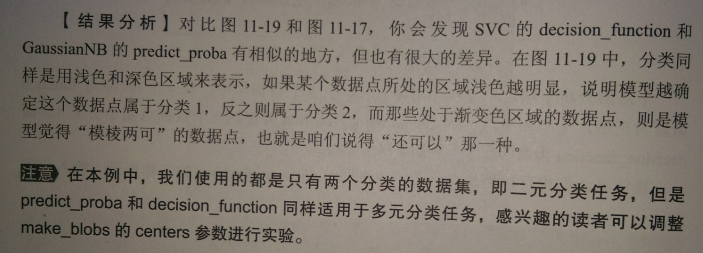.score给模型评分的方法

• 对于分类模型来说，默认情况下，.score给出的是模型分类的准确率
• 对于回归模型来说，默认情况下， .score给出的是回归分析中的R2分数【即，可决系数或拟合优度】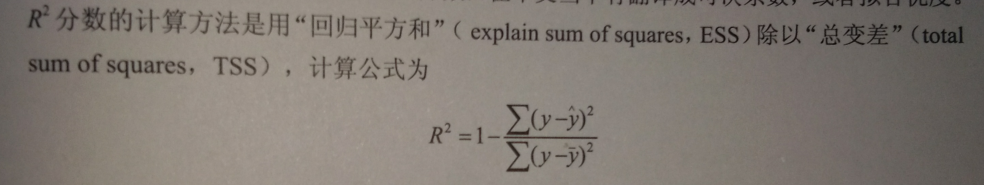其他评分的方法：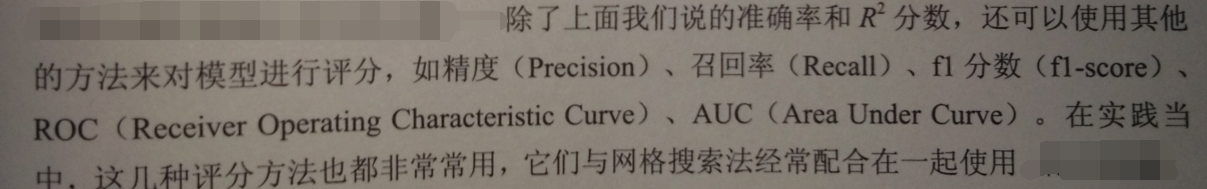GridSearchCV改变评分的方式：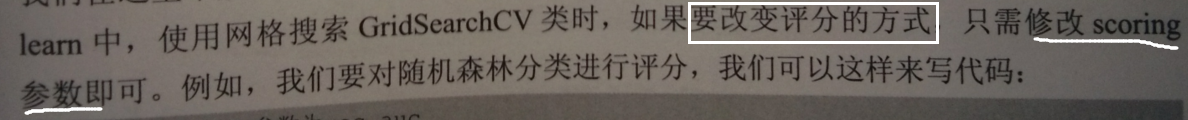#修改scoring参数为roc_auc
grid = GridSearchCV(RandomForestClassifier*(,param_grid = param_grid,scoring = 'roc_auc')

posted @ 2019-05-07 21:21  远征i  阅读(920)  评论(0编辑  收藏